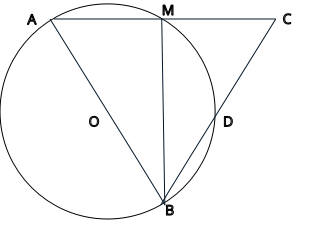### Prove: Triangle AMB is congruent to Triangle CMB<AMB = 90
Substitution
<ABM = (1/2) * arc AM and <CBM = (1/2) * arc DM
<ABM = <CBM
<CMB = 90
Inscribed angle measures half its intercepted arc
Circle O with diameter AB, arc AM = arc DM
Substitution
The angles in a linear pair are supplementary
Given
arc AB = 180
Triangle AMB is congruent to Triangle CMB
ASA (Angle-Side-Angle)
Reflexive
Inscribed angle measures half its intercepted arc
MB = MB
<AMB = (1/2) * arc AB
Definition